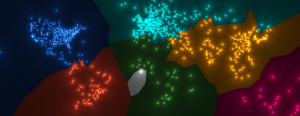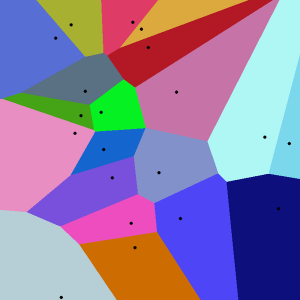Have you ever wondered what the IP distribution looks like ?When it comes to network security research, we usually find ourselves looking at wide IP address sets. Often, we’re interested in understanding the distribution of the IP addresses we are looking at in terms of geolocation as well as IP prefixes/ranges. For example, in our studies of the Kelihos botnet or the Cryptolocker ransomware, we can gather a list of the infected client IPs and observe the impact of the infection. It definitely helps us appreciate the magnitude and the nature of the problem.

Before we go into more details and interpretations, let’s introduce our layout technique and some important facts about the Internet.

## Geometric concept

#### IP to 2D space

An IPv4 address is made of 4 natural numbers between 0 and 255. In the mathematical sense, it can be seen as a 4-dimensional integer vector.

[I = left ( mathbb{Z} cap [0, 255] right )^{4}]

Now, the whole idea is to build a function f to transform this IP into a 2-dimensional real vector. Then if we take the whole IP set and apply this transformation to every IP, we will build a 2D point cloud that will represent our IP set.

[f : I rightarrow mathbb{R}^{2}]

In other words, it is a projection of the IP space into the [mathbb{R}^{2}] space. There are many ways to do so—today we’re going to present a few approaches. But first, let’s rescale our IP addresses to be a bit more manipulable.

[Let i in I,  i = [A, B, C, D]\Let j = frac{1}{255}i = frac{1}{255}[A, B, C, D] = [a, b, c, d]]

Great! Now we have an IP address (a, b, c, d) where every component is rescaled between 0 and 1.

Now let’s define our transformation function:

[f(a,b,c,d) = left{begin{matrix}(r + wb)cos alpha + r’dcosgamma\(r + wb)sin alpha + r’dsingamma\end{matrix}right.]

with

[left{begin{matrix}r = 32 rightarrow inner radius\ r’ = 0.5 rightarrow disk radius\ w = 255 rightarrow arc width \ end{matrix}right.]

and

[left{begin{matrix}alpha = frac{7pi a}{4}-frac{3pi}{8} rightarrow arc angle\gamma = 2pi c rightarrow disk angle\\end{matrix}right.]

These formulas may scare you if you haven’t done trigonometry in a while but it is actually very simple. The function can be split in two parts :

[f(a,b,c,d) = left{begin{matrix}(r + wb)cos alpha\(r + wb)sin alpha \end{matrix}right.+left{begin{matrix}r’dcosgamma\r’dsingamma\end{matrix}right.]

The first part is the definition of our arc using polar coordinates. We use an inner radius and a width variable to control its shape. The second part is the definiton of a disk in polar coordinates as well. What we have here is the definition of two systems: one using A and B to find a position in the arc given a certain inner radius and width, and the other one using C and D to find a position around the former point given a certain disk radius. A and C are rescaled on the trigonometrical circle to define angles, B and D are used as distances from the origin (radius).

In simpler words :

• The arc represents the whole IP space (center right is 0.0.0.0, center left is 255.255.255.255)
• A line from the center represents an A.*.*.* IP range
• A point in the arc represent an A.B.*.* IP range
• A bright point represents a denser /16 prefix in A.B.*.*
• A dim point represents a sparser /16 prefix in A.B.*.*

#### Coloring

Now we have a point cloud built from an IP set, how do we color it ? First we give a color to every point. In our case, the color represents either a continent code or a country code. It could be something completely different. Then, for each pixel of the texture, we search for the nearest point in the cloud and take its color. This structure is also known as a Voronoi diagram, and it’s heavily used in area analysis and computational geometry. In our case, we’ll use it to represent continent or country territories.

Here is what a Voronoi diagram looks like :To obtain the final result, we need one last thing. We scale the pixel color relatively to its distance to its nearest point to achieve the gradient effect.
Note, however, that we don’t necessarily have to use the Euclidian distance for our purpose; different distance functions will give different outputs (Ex: Manhattan distance).

In our case we use the logarithm of the euclidian distance:

[Let overrightarrow{P} be our current pixel position\ Let overrightarrow{N} be the nearest point position\Let overrightarrow{D} be overrightarrow{P}-overrightarrow{N}]
[tint(overrightarrow{D}_{x,y}) = frac{1}{log(1 + sqrt{x^{2} + y^{2}} + varepsilon) } with varepsilon > 0\\color(overrightarrow{P}) = tint(overrightarrow{D}) * overrightarrow{N}_{color}]

## Internet territories

Now that we have our geometrical model ready to go, we would like to use it on a couple of datasets. But before we do that we need to focus on a very important point in order to make an accurate diagnosis.

Consider the two pictures below : The first one is a continent view (Colors are Continent Codes), the second is a country view (Colors are Country Codes)

These pictures have been created by generating 10000 random IP addresses with a uniform distribution :

def populate_random_ips(count):

    for i in range(count):        a = random.choice(range(0, 255))         b = random.choice(range(0, 255))        c = random.choice(range(0, 255))        d = random.choice(range(0, 255))
        load_ip(str(c) + "." + str(d) + "." + str(a) + "." + str(b))

Then, we use the GeoIP library to get a continent and country code. We associate one color for each code and run the same layout process.

NOTE : The bright white color represent the reserved IP areas and the grey color represents the values that don’t have a continent or country code.

Remarks / Interpretations :

• The bright white color represents the reserved IP ranges :
• 10.0.0.0/8, 172.16.0.0/12, 192.168.0.0/16 : Local communications within a private network
• 240.0.0.0/4 : Reserved for future use
• 255.255.255.255/32 : Reserved for limited broadcast
• Etc. For more details on the reserved address spaces : http://en.wikipedia.org/wiki/Reserved_IP_addresses
• Some continents occupy much bigger/smaller areas in the IP space than others.
• Asia (Yellow) and Europe (Light blue) split into dozens of countries from the continent to the country view.
• Africa (green), South America (red) and Oceania (pink) are clearly less predominant in the IP space.
• We can see the IP frontiers in isolated points. Some pink points (Oceania) inside the big yellow space (Asia) represent Indonesia and some yellow points in the light blue (Europe) area represent Eastern Europe.

To summarize, it is a well known fact that the IP distribution is obviously not uniform and we need to keep this crucial fact in mind to appreciate the next datasets.

## IP Infection maps

Now let’s present a couple of infected IP sets gathered by the Research Team and let’s see some interpretations.

The first picture is the continent view, the second is the country view. Note that the color code is not necessarily the same in each country view.

#### 1. Cryptolocker (843 IPs)

This IP set is a list made by our researcher Ping of all the client IPs infected by the Cryptolocker ransomware in one single day (Oct 30th)

Interpretation

The infected clients are mostly located in North America and Europe. The first thing we notice is that the continent view and country view look pretty similar, in the global IP map (first picture of this blog), we can see that the big light blue range splits into many different countries which is interestingly not the case here since the ransomware has predominantly infected machines in the UK. And finally, we can see that the US was the most infected country.

#### 2. Weight Loss Scam (6049 IPs)

This IP set is a list our researcher Frank made of IP addresses hosting malicious content (Weight Loss Scam) taken from July 7th to today.

Interpretation :

Here we can clearly see a relatively strong presence in South America,  Africa. Given the small proportion they represent on the IP world map (First picture of this blog) the vivid green and red colors are noticeable. The machines are spread out in Europe and Asia have been infected  (Notice the light blue and yellow subdivision into many different colors in the country view) and also very present in the US.

#### 3. Kelihos (42244 IPs)

This IP set is a list made by our researcher Dhia representing all the client IPs infected by the Kelihos botnet.

For more details on how Dhia built this dataset, check out our Kelihos blog posts (1) and (2).

This one is clearly amazing. Since it contains more than 40,000 IPs, we would have expected a wide distribution but this is not the case here. This means the Kelihos botnet has infected a lot of sub networks and /16 prefixes. We notice a very strong presence in Europe, Asia and more precisely Eastern Europe (yellows in light blues). Almost nothing in Oceania (a single pink dot) and also a weak presence in the US (given the proportion of IPs it represents).

#### Conclusion

To conclude, we presented an interesting way to correlate geolocations and IP addresses of various datasets using some trigonometry and graphics knowledge. In further experiments, it would be interesting to use the colors to visualize something else (Ex : ASN, IP reputation score etc.). Also, since this layout is using only 2 dimensions, one way to improve it is to use the third dimension to represent other metrics (Ex : Traffic, DNS requests etc.).